DraftKings 2019 ACC Odds
+250
2.5 to 1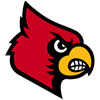Louisville
21.8% implied probability

+300
3 to 1Duke
19.0% implied probability

+300
3 to 1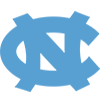North Carolina
19.0% implied probability

+450
4.5 to 1Virginia
13.8% implied probability

+1000
10 to 1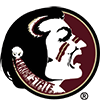Florida State
6.9% implied probability

+2500
25 to 1North Carolina State
2.9% implied probability

+2500
25 to 1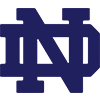Notre Dame
2.9% implied probability

+2500
25 to 1Virginia Tech
2.9% implied probability

+3500
35 to 1Clemson
2.1% implied probability

+3500
35 to 1Syracuse
2.1% implied probability

+4000
40 to 1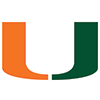Miami
1.9% implied probability

+5000
50 to 1Pittsburgh
1.5% implied probability

No changes have been recorded yet.
+6600
66 to 1Boston College
1.1% implied probability

No changes have been recorded yet.
+6600
66 to 1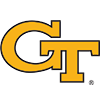Georgia Tech
1.1% implied probability

No changes have been recorded yet.
+10000
100 to 1Wake Forest
0.8% implied probability